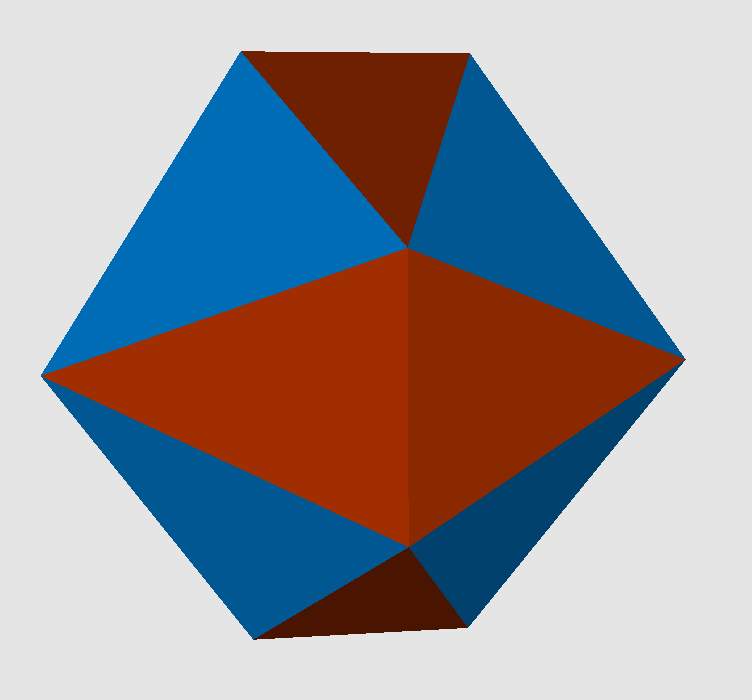Acronym snit Name pyritohedron,pyritosnub tetrahedron,o2o2o symmetric ike variant,vertex figure of hyperbolic cube + n-prism honeycomb `` Circumradius sqrt[a2+c2] Especially cube-dim-doe (c:a = F)   alternated toe (a,c,d) = (q,Q,h) Confer uniform variants: ike (c:a = f)   co (c:a = 1)   general polytopal classes: isogonal ExternallinksThis polyhedron is isogonal only. It requires for 2 edge sizes a and d. (c here is a pseudo edge only.)

The transition a → c will result in qqo oqq qoq&#zx (co). Then the a edges also become pseudo ones too, the incidences then would differ accordingly.

The case d:a = 1, c:a = f results in the fxo xof ofx&#zx (ike).

The case (a,c,d)(q,Q,h) (where Q = 2q, i.e. c:a = 2) results in the Qqo oQq qoQ&#zh (alternated toe) and would reflect the mere alternated faceting wrt. a vertex alternation with starting figure toe.

The case d:a = f, c:a = F results in the Fxo xoF oFx&#zf (cube-dim-doe).

Incidence matrix according to Dynkin symbol

```cao aoc oca&#zd   → height = 0
a < c
d = sqrt[(a2-ac+c2)/2]

o.. o.. o..     & | 12 | 1  4 |  3 2
------------------+----+------+-----
... a.. ...     & |  2 | 6  * |  2 0  a
oo. oo. oo.&#d  & |  2 | * 24 |  1 1  d
------------------+----+------+-----
... ao. ...&#d  & |  3 | 1  2 | 12 *  add
ooo ooo ooo&#d    |  3 | 0  3 |  * 8  d-{3}
```